StatLect

# Computing the Riemann-Stieltjes integral: some rules

In the lecture on the Expected value we have discussed a rigorous definition of expected value that involves the Riemann-Stieltjes integral. We present here some rules for computing the Riemann-Stieltjes integral. Since we are interested in the computation of the expected value, we focus here on rules that can be applied when the integrator function is the distribution function of a random variable, that is, we limit our attention to integrals of the kind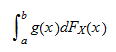whereis the distribution function of a random variableand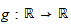. Before stating the rules, note that the above integral does not necessarily exist or is not necessarily well-defined. Roughly speaking, for the integral to exist the integrand functionmust be well-behaved. For example, ifis continuous on, then the integral exists and is well-defined.

That said, we are ready to present the calculation rules:

1.is continuously differentiable on. Ifis continuously differentiable onandis its first derivative, then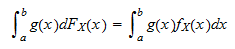2.is continuously differentiable onexcept at a finite number of points. Supposeis continuously differentiable onexcept at a finite number of points, ...,such thatDenote the derivative of(where it exists) by. Then,1. Exercise

## Exercise

Letbe defined as follows: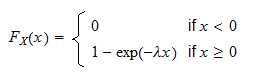where.

Compute the following integral: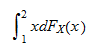Solutionis continuously differentiable on the interval. Its derivativeisAs a consequence, the integral becomes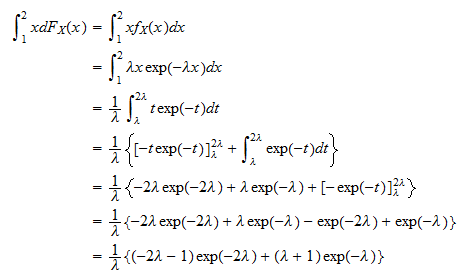The book

Most of the learning materials found on this website are now available in a traditional textbook format.

Glossary entries
Share